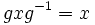# Conjugacy class

## Contents

VIEW RELATED: Analogues of this | Variations of this | Opposites of this |[SHOW MORE]

## Definition

### Symbol-free definition

A conjugacy class in a group can be defined in any of the following ways:

### Definition with symbols

Suppose$G$ is a group. A conjugacy class in$G$ is a nonempty subset$c$ of$G$ such that the following two conditions hold:

• Given any$x,y \in c$, there exists$g \in G$ such that$gxg^{-1} = y$.
• If$x \in c$ and$g \in G$ then$gxg^{-1} \in c$.

In other words, it is closed under the action of the group on itself by conjugation, and the action is transitive when restricted to the conjugacy class. In the language of group actions, it is a single orbit under the action of the group on itself by conjugation.

Any group is a disjoint union of conjugacy classes.

## Examples

If you're interested in conjugacy classes in a particular group, go to the article on that group, and look at the subsection titled Under conjugation in the section titled Elements.
• In an Abelian group, all the conjugacy classes are singleton sets of size 1. That's because for any$x,g$, we have$gxg^{-1} = x$
• More generally, for any group$G$, and any element in the center of$G$, the conjugacy class of that element has size 1.
• The symmetric group on three letters is the smallest non-Abelian group. This group has three conjugacy classes, the class of the identity element (size 1), the class of the transpositions (size 3) and the class of the 3-cycles (size 2).
• More generally, in the symmetric group on any set, two permutations are in the same conjugacy class if and only if they have the same cycle type. Further information: Cycle type determines conjugacy class

## Facts

• The size of any conjugacy class divides the order of the group, for a finite group. More precisely, it is the index of the centralizer of any element of the conjugacy class. This follows from the more general fact that the size of any orbit under a group action is the index of the stabilizer of any point in the orbit. Further information: size of conjugacy class divides order of group, size of conjugacy class divides index of center
• A conjugacy class has size 1 iff the corresponding element is in the center.
• The sizes of the conjugacy classes are related by a class equation
• The set of conjugacy classes can be given an algebraic structure in various ways; for instance, the convolution algebra on conjugacy classes
• For a finite group$G$, given a conjugacy class$c$ and a natural number$n$ which divides the order of the group, the number of solutions to$x^n \in c$ is a multiple of$n$